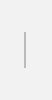# Modular multiplication

## Explanation

The integer remainder of the multiplication x*y divided by n, is written as "x*y mod n". Such an operation is called modular multiplication and the integer n is called the modulus. In calculation of RSA or HIME(R), n is a composite number, i.e., two or more prime numbers is used for composite number.